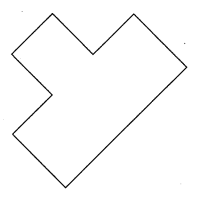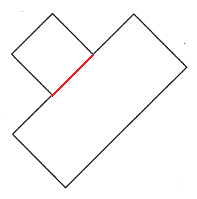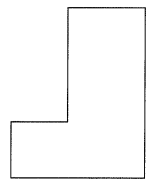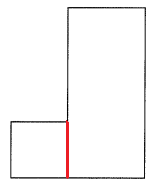# Math in Focus Grade 4 Chapter 11 Practice 1 Answer Key Squares and Rectangles

This handy Math in Focus Grade 4 Workbook Answer Key Chapter 11 Practice 1 Squares and Rectangles detailed solutions for the textbook questions.

### Math in Focus Grade 4 Chapter 11 Practice 1 Answer Key Squares and Rectangles

Fill in the blanks with yes or no.

Example
Is this a square? yes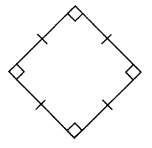Question 1.
Is this a rectangle? ____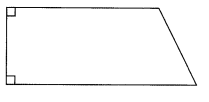No, The above figure is not a rectangle.

Question 2.
Is this a square? ____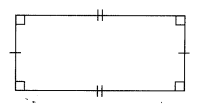No, The above figure is not a square.

Question 3.
Is this a rectangle? ___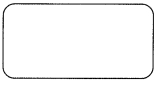No, The above figure is not a rectangle.

Fill in the blanks.

Example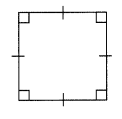Is this a square? Yes
Why or why not? All its sides are of equal length, and it has four right angles.

Question 6.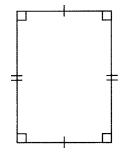Is this a rectangle? ____
Why or why not? ________
Yes
A rectangle is a type of quadrilateral that has its parallel sides equal to each other and all the four vertices are equal to 90 degrees. Hence, it is also called an equiangular quadrilateral.

Question 7.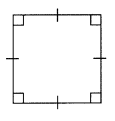Is this a rectangle? ____
Why or why not? ________
No
A Rectangle is a four sided-polygon, having all the internal angles equal to 90 degrees. The two sides at each corner or vertex, meet at right angles. The opposite sides of the rectangle are equal in length which makes it different from a square. The above figure is a square because all sides are of equal length.

Question 8.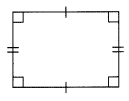Is this a rectangle? ____
Why or why not? ________
Yes
A rectangle is a type of quadrilateral that has its parallel sides equal to each other and all the four vertices are equal to 90 degrees. Hence, it is also called an equiangular quadrilateral.

Find the lengths of the unknown sides.

Example
ABCD is a square.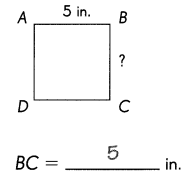Question 9.
EFGH is a rectangle.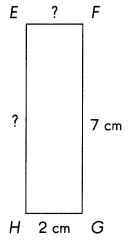EF = ______ cm
EH = ______ cm
EF = 2 cm
EH = 7 cm
Explanation:
In the above image we can observe a rectangle of EFGH. A rectangle is a type of quadrilateral that has its parallel sides equal to each other and all the four vertices are equal to 90 degrees.

Question 10.
PQRS is a square.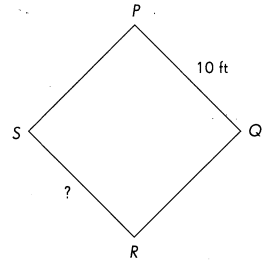SR = __ ft
SR = 10 feet
Explanation:
In the above image we can observe a square of PQRS. A square is a  plane figure with four equal straight sides and four right angles.

Question 11.
ABCD is a rectangle.
Its length is twice its width.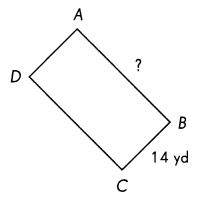AB = __ yd
Width = 14 yards
Length = 2 x width
= 2 x 14
= 28 yards
AB = 28 yards
Explanation:
In the above image we can observe a rectangle ABCD. A rectangle is a type of quadrilateral that has its parallel sides equal to each other and all the four vertices are equal to 90 degrees.

Draw a line segment to break up each figure into two rectangles.

Example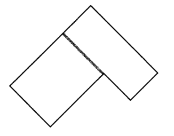Question 12.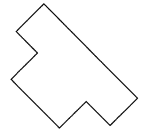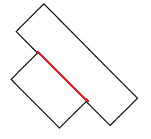Explanation:
To break above figure into two rectangles we have to draw one line segment. The line segment is represented with red color as we can observe in the above figure.

Question 13.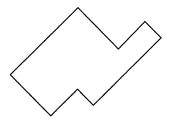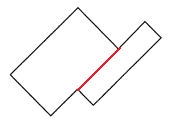Explanation:
To break above figure into two rectangles we have to draw one line segment. The line segment is represented with red color as we can observe in the above figure.

Question 14.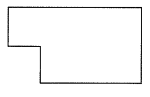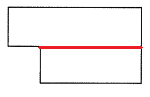Explanation:
To break above figure into two rectangles we have to draw one line segment. The line segment is represented with red color as we can observe in the above figure.

Draw a line segment to break up each figure into one square and one rectangle.

Question 15.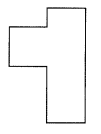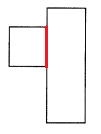Explanation:
To break above figure into one square and one rectangle we have to draw one line segment. The line segment is represented with red color as we can observe in the above figure.

Question 16.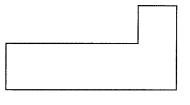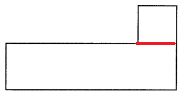Explanation:
To break above figure into one square and one rectangle we have to draw one line segment. The line segment is represented with red color as we can observe in the above figure.

Question 17.